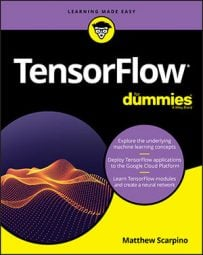##### TensorFlow For DummiesMachine learning applications frequently need exponents and logarithms to compute errors and probability. To meet this need, TensorFlow provides many of the same functions available in NumPy. The following table lists 11 of them and provides a description of each.

Exponential and Logarithmic Operations

 Function Description `square(x, name=None)` Returns the square of the argument `squared_difference(x, y, name=None)` Subtracts the first argument from the second and returns the square `sqrt(x, name=None)` Returns the square root of the argument `rsqrt(x, name=None)` Returns the reciprocal of the square root `pow(x, y, name=None)` Returns elements of the first tensor raised to the power of the elements of the second vector `exp(x, name=None)` Returns the exponential function of the argument `expm1(x, name=None)` Returns the exponential function of the argument minus one, exp(x) - 1 `log(x, name=None)` Returns the natural logarithm of the argument `log1p(x, name=None)` Returns the natural logarithm of the argument plus 1, log(x + 1) `erf(x, name=None)` Returns the error function of the argument `erfc(x, name=None)` Returns the complementary error function of the argument
These functions are straightforward to use and understand. Each executes in an element-wise manner, and the following code demonstrates how you can call `square`, `sqrt`, and `rsqrt`:
`t = tf.constant([4.])t1 = tf.square(t)                # 16t2 = tf.sqrt(t)                  # 2t3 = tf.rsqrt(t)                 # 0.5`
The `exp `function computes the exponential functions of a tensor's elements, and expm1 subtracts 1 from each exponential. If x is a value in the input tensor, the result of `expm1 `equals `exp(x) – 1`.

Similarly, the log function computes the natural logarithm of a tensor's elements. `logp1 `adds 1 to the value before the logarithm is computed, so if x is a value in the input tensor, the result of `logp1 `equals `log(x + 1)`.